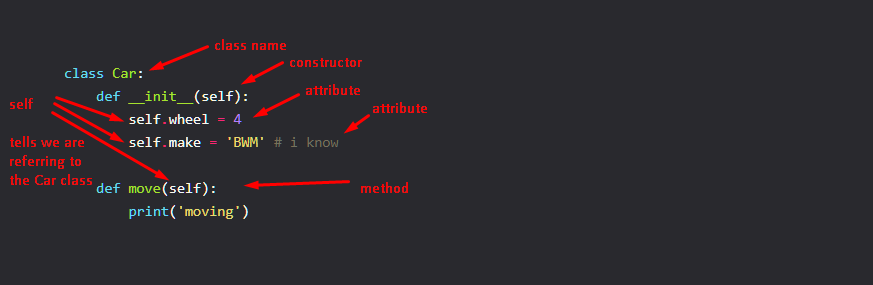In Python, you can use functions as classes. In py, everything is an object. How? I'm no py expert. Here's how we do it!

## An Innocent Python Function

a function is like that

```def devdotto():
pass
```

and a class is like that:

```class Car:
def __init__(self):
self.wheel = 4
self.make = 'BWM' # i know

def move(self):
print('moving')
```make and wheels are attributes while move is a method

some tests

```car = Car()

print(car.wheel)
print(car.make)

car.move()
```

gives out

```4
BWM
moving
```

## Now Let Us Add Attributes

```devdotto.name = 'dev.to'
devdotto.users = 1123234
```

testing

```print(devdotto.name)
print(devdotto.users)
```

gives out

```dev.to
1123234
```

## More Craziness: Adding Methods To Functions

```devdotto.add = lambda x,y: x+y
```

some info:testing

```print(devdotto.add(1,2))
```

gives out

```3
```

## Conclusion

Since some one liner libs make use of IEF (Immediately Executed Functions) using lambdas to compile even loops, these can be useful, but i can't think of any use cases (for using functions as classes), this said, this is one of py's party tricks, hence the cover image.